• 作图存在汉字无法显示 在作图代码前添加如下代码 plt.rcParams['font.sans-serif...plt.plot()中有一个参数 ‘linewidth’，修改参数可以改变曲线粗细程度 一个坐标系中存在多个曲线，给曲线命名 plt.plot()中有一个...
作图存在汉字无法显示
在作图代码前添加如下代码
plt.rcParams['font.sans-serif'] = ['SimHei']
plt.rcParams['axes.unicode_minus'] = False

作图的曲线粗细调整
plt.plot()中有一个参数 ‘linewidth’，修改参数可以改变曲线粗细程度
一个坐标系中存在多个曲线，给曲线命名
plt.plot()中有一个参数 ‘label’，对应该函数画出的曲线
使用plt.plot()设定了label后并未画出
在后面添加如下代码
plt.legend()

坐标轴label
font = {'family': 'Times New Roman', 'weight': 'normal', 'size': 23}
plt.xlabel('x position', font)
plt.ylabel('y position', font)

给定坐标系长度
将坐标系固定为横坐标0 ~ 100，纵坐标0 ~ 350
plt.axis([0, 100, 0, 350])

坐标系名字
font = {'family': 'Times New Roman', 'weight': 'normal', 'size': 23}
plt.title('name', font)

坐标系标度字体大小
plt.xticks(fontsize=20)
plt.yticks(fontsize=20)



展开全文作图
• plt.xticks()第一个参数接受坐标，第二个参数接受，各坐标显示的文本，关键字参数，如 rotation，表示文本显示时旋转的角度，为了达到一种美观的效果。 import matplotlib.pyplot as plt import numpy as npif __...
plt.gca().grid(True)：显示网格线；
plt.colorbar()：显示颜色条；
0.  可选参数

plt.imshow()

cmap：plt.cm.binary（二值图）/plt.cm.gray（灰度图）/plt.cm.bone/plt.cm.hot
绘图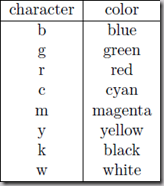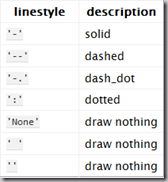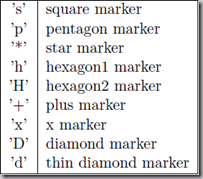1. plt.xticks()

第一个参数接受坐标，第二个参数接受，各坐标显示的文本，关键字参数，如 rotation，表示文本显示时旋转的角度，为了达到一种美观的效果。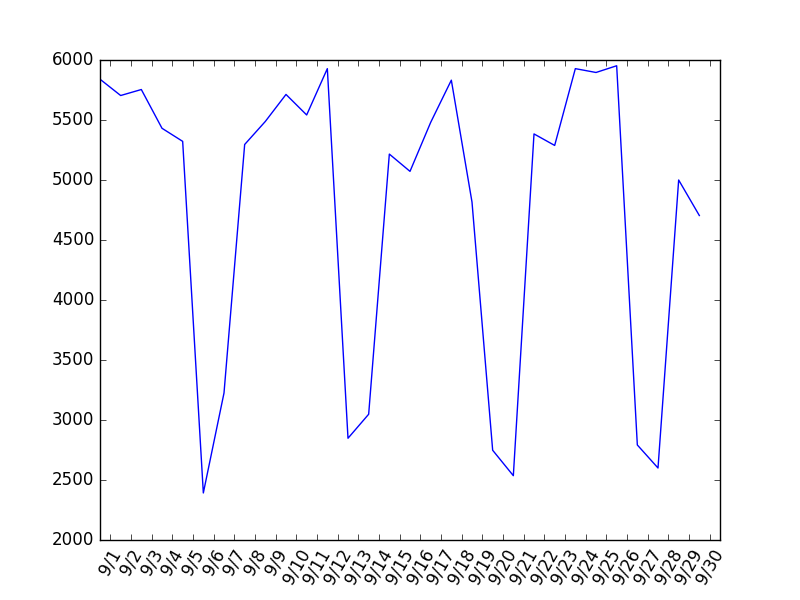import matplotlib.pyplot as plt
import numpy as np

if __name__ == '__main__':
data = [5840, 5704, 5754, 5431, 5322, 2392, 3225, 5296, 5488, 5713, 5542, 5928, 2848, 3048, 5216,
5072, 5480, 5832, 4816, 2748, 2536, 5384, 5288, 5928, 5896, 5952, 2792, 2600, 5000, 4704]
plt.plot(data)
width = 1.
plt.xticks(np.arange(len(data))+width/2, ['9/'+str(i) for i in range(1, 31)], rotation=60)
plt.show()
展开全文• matplotlib.pyplot.plot()参数详解 plt.plot(x,y,format_string,**kwargs) x轴数据，y轴数据，format_string控制曲线的格式字串。 format_string 由颜色字符，风格字符 和 标记字符 组成。参数是一个可变长度...
原文出处：

Python的知识点 plt.plot()函数细节

matplotlib.pyplot.plot()参数详解

plt.plot(x,y,format_string,**kwargs)

x轴数据，y轴数据，format_string控制曲线的格式字串。

format_string 由颜色字符，风格字符 和 标记字符 组成。参数是一个可变长度参数。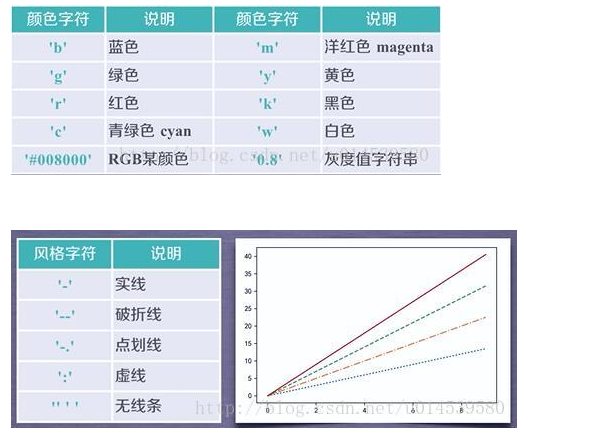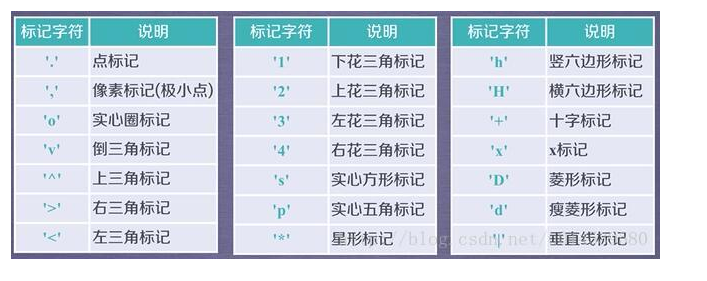关于*kwargs，有时候，函数的参数里会有(*args, *kargs)，都是可变参数，*args表示无名参数，是一个元租，**kwargs是键值参数，相当于一个字典，比如你输入参数为：(1,2,3,4,k,a=1,b=2,c=3)，*args=(1,2,3,4,k)，**kwargs={'a':'1,'b':2,'c':3}。

例如，下面的每一个都是合法的：

plot(x, y)       #plot x, y使用默认的线条样式和颜色

plot(x, y, 'bo')    #plot x,y用蓝色圆圈标记

plot(y)    #plot y用x作为自变量

plot(y, 'r+')      #同上，但是是用红色作为标记

更多参数：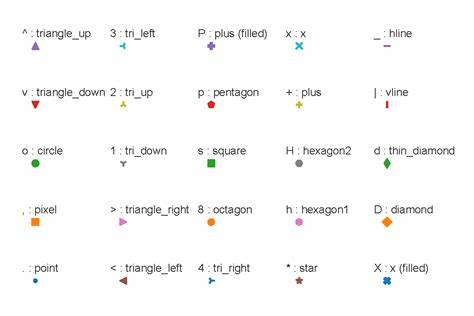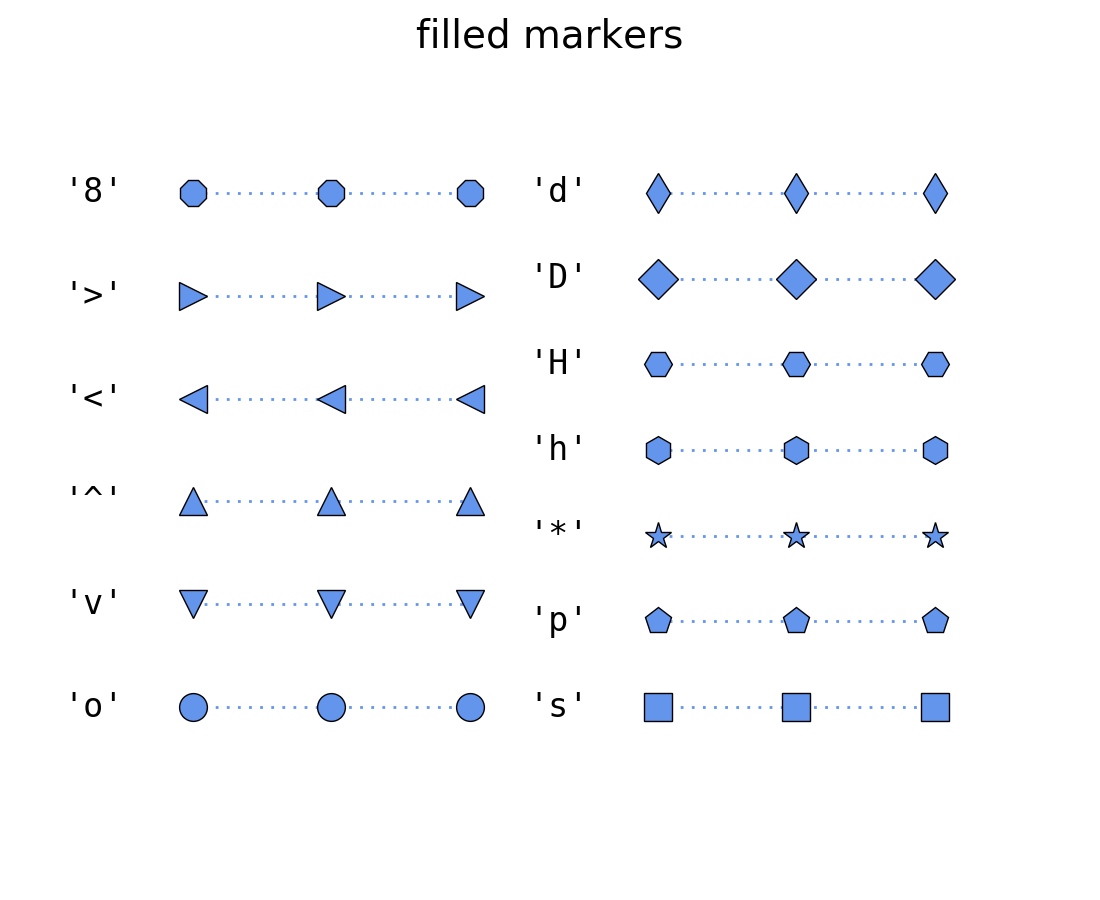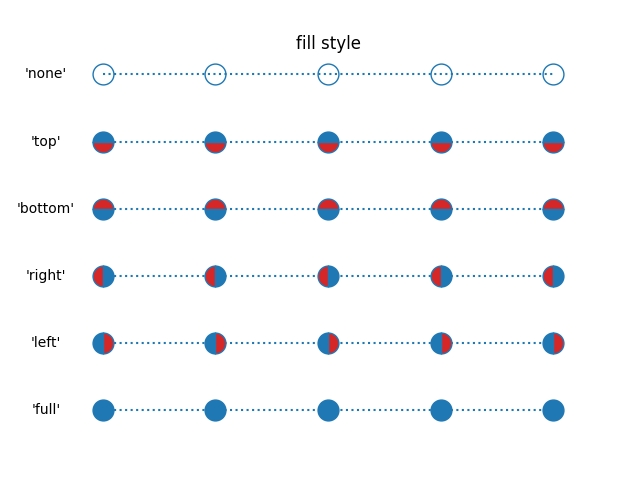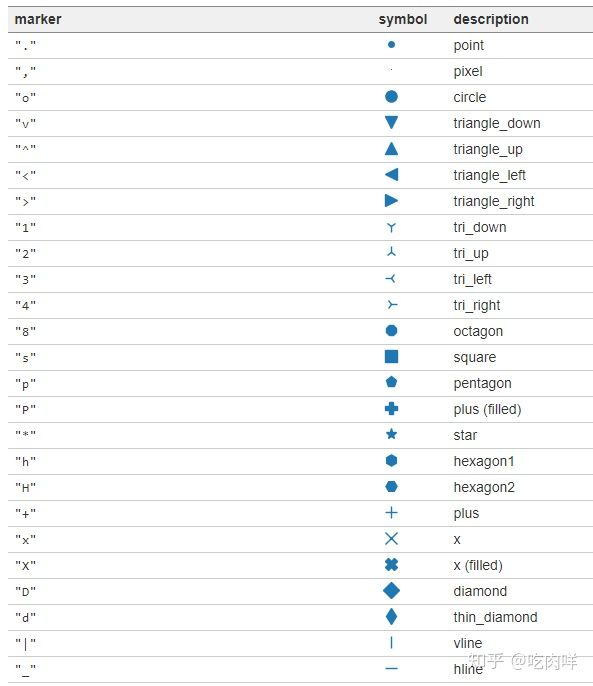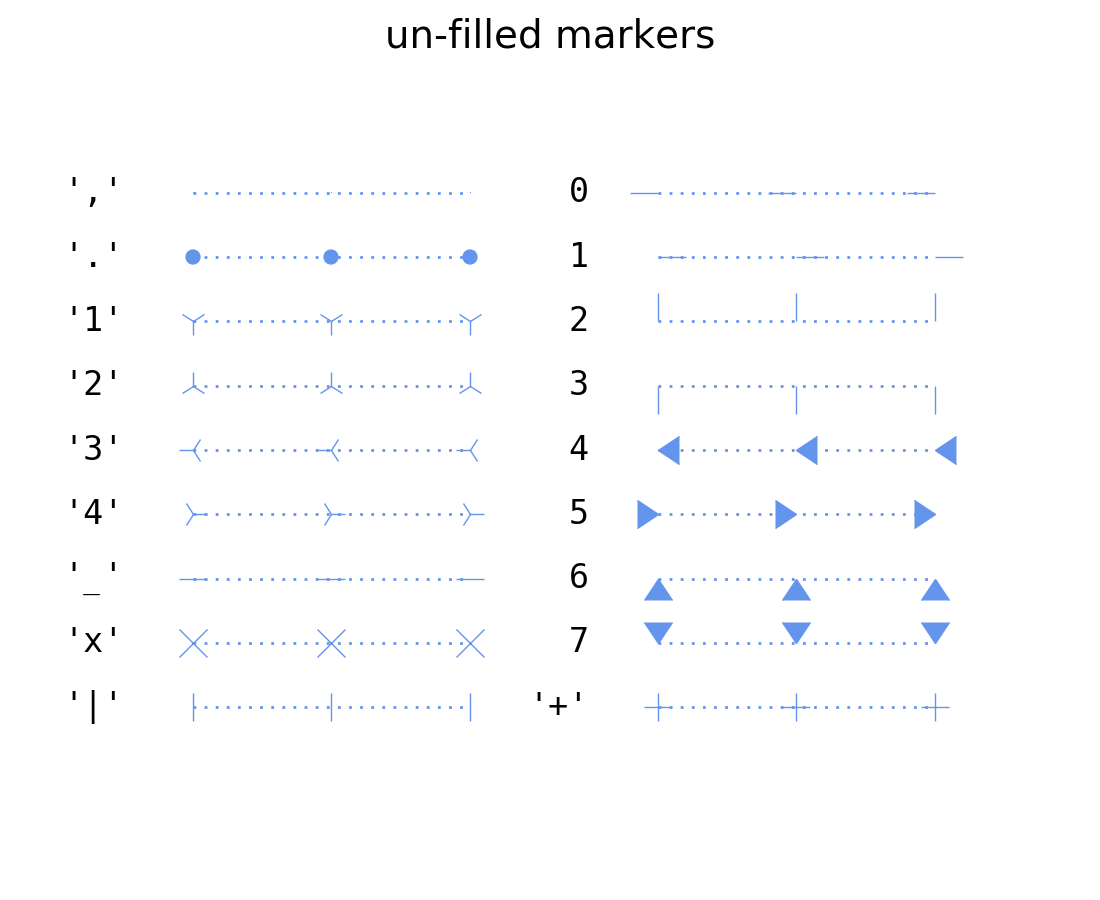展开全文• pyplot是替代matlab的一个很好的选择，不过默认的曲线绘图不够美观，这里对一些细节参数进行了修改，以供各位参考。 2. 绘图效果对比 修改前（右图）与修改后（左图）对比 注：图中曲线数据来源是对文献 ...
1. 概述

pyplot是替代matlab的一个很好的选择，不过默认的曲线绘图不够美观，这里对一些细节参数进行了修改，以供各位参考。

2. 绘图效果对比

修改前（右图）与修改后（左图）对比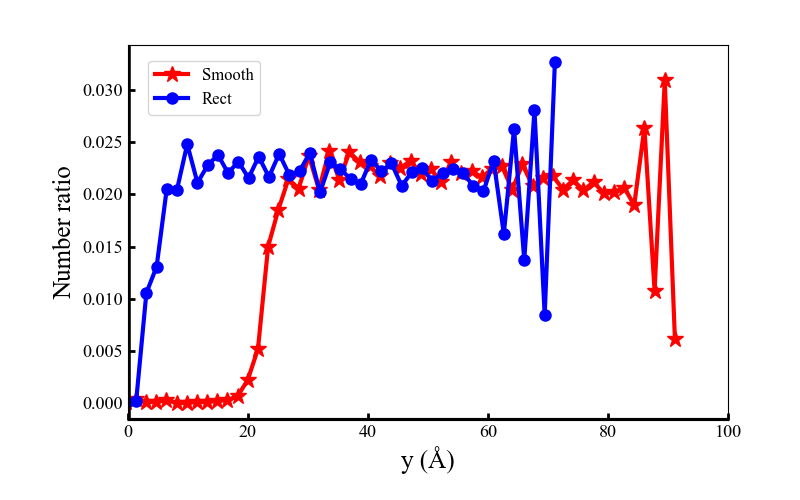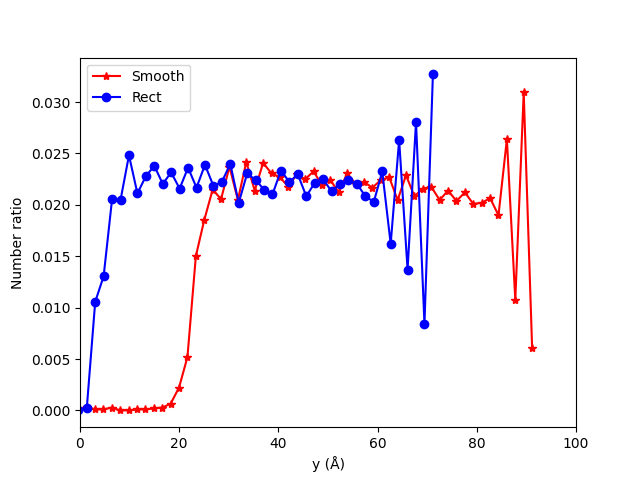注：图中曲线数据来源是对文献

Hu Chengzhi, Tang Dawei, Lv Jizu, et al. Molecular dynamics simulation of frictional properties of Couette flow with striped superhydrophobic surfaces under different loads.. 2019, 21(32):17786-17791.

的复现与学习，感兴趣的同学可以阅读原文。

3. 修改前后代码

注：以下示例程序中不包含数据，不可直接运行。

3.1 导入库

import matplotlib.pyplot as plt
import matplotlib as mp

3.2 修改前

plt.figure()
plt.plot(d_plot1[:, 0]-8, d_plot1[:, 1], label='Smooth',color='r',marker="*")
plt.plot(d_plot2[:, 0]-8, d_plot2[:, 1], label='Rect',color='b',marker="o")
plt.xlabel(xlabel='y (Å)')
plt.ylabel(ylabel='Number ratio')
plt.legend()
plt.xlim((0, 100))
plt.show()

3.3 修改后

plt.figure(figsize=(8, 5))                                                     # 打开指定大小的窗口
plt.axes([0.16, 0.16, 0.75, 0.75])                                             # 偏移绘图区域，从而完整显示坐标轴名称
plt.plot(d_plot1[:, 0]-8, d_plot1[:, 1], label='Smooth',color='r', linewidth=3, marker="*", markersize= 12)
plt.plot(d_plot2[:, 0]-8, d_plot2[:, 1], label='Rect',color='b', linewidth=3, marker="o", markersize= 8)
#                                        图例名称        颜色        曲线线宽       标记符号     标记符号尺寸
plt.xticks(fontsize= 13, fontfamily= "Times New Roman")                        # x 轴刻度字体大小，字体类型
plt.yticks(fontsize= 13, fontfamily= "Times New Roman")                        # y 轴刻度字体大小，字体类型
plt.tick_params(direction= "in", width= 2.0, length= 5.0)                      # 刻度显示在绘图区域内侧，宽度2.0，高度5.0
plt.axhline(y=plt.ylim(), xmin= 0.0, color= "k", linewidth= 3.0)            # 加粗显示 x 轴
plt.axvline(x=plt.xlim(), ymin= 0.0, color= "k", linewidth= 4.0)            # 加粗显示 y 轴
plt.xlabel(xlabel='y (Å)', fontsize= 18, fontfamily= "Times New Roman")        # x轴名称及字号字体
plt.ylabel(ylabel='Number ratio', fontsize= 18, fontfamily= "Times New Roman") # y轴名称及字号字体
# plt.ylabel(ylabel='Friction force(eV/A)', fontsize= 18, fontfamily= "Times New Roman")
legend_font= mp.font_manager.FontProperties(family="Times New Roman", size= 12)# 定义字体
plt.legend(prop= legend_font,loc='upper left', bbox_to_anchor=(0.02, 0.98))    # 图例字体
# plt.savefig('temp.png')                                                      # 保存图片
plt.xlim((0, 100))                                                             # 设置显示范围
plt.show()                                                                     # 显示曲线


展开全文• 1、plt.plot(x,y,format_string,**kwargs) ...format_string 由颜色字符，风格字符，和标记字符 关于*kwargs，有时候，函数的参数里会有(*args, *kargs)，都是可变参数，*args表示无名参数，是一个元...
• 文章目录1. 绘制散点图1.1 将散点图着色1.2 将散点图进行颜色映射 cmap1.3 设置图...import matplotlib.pyplot as plt x = list(range(1,50)) y = [i**2 for i in x] plt.scatter(x,y,s=40) //这里前两个参数分别是x
• 设置坐标轴 ​ 还记得上次画的那条“项链”嘛？结尾的时候有说过，这些是新手村礼包，还有很多其他值得探索的地方呢，那么就一起来康康还有...不要太在意细节哈，忽视那个长的不太协调的x轴和弯弯曲曲如蚯蚓的抛物线可视化 数据可视化 python
• 由于matplotlib是一个专业，工业化的图形库，它对很多细节性的功能也作了深入的定制，让它满足各种各样的需求。在本文里就介绍一下怎么样来设置曲线的点划线的样式，如下图： 像上图就有很多类型的点划线，在...python 可视化
• 在做完数据分析后,有时候需要将分析结果一目了然地展示出来,此时便离不开Python可视化工具,Matplotlib是Python中的一个2D绘图工具,是另外一...图形细节调整(这里可以做很多调整,如x/y轴文字参数说明,颜色/线粗/柱状...
• 3.图形细节调整(这里可以做很多调整,如x/y轴文字参数说明,颜色/线粗/柱状粗度,x/y轴文字角度等) 4.显示图像(调用show()) 总结下一个区域同时绘制多个子图的步骤 1.确定绘图区域大小 2.确定每个子图在绘图区域的位置 ...
• 在做完数据分析后,有时候需要将分析结果一目了然地展示出来,此时便离不开Python可视化工具,Matplotlib是Python中的一个2D绘图工具,是...3.图形细节调整(这里可以做很多调整,如x/y轴文字参数说明,颜色/线粗/柱状粗度,
• 同样，如果使用函数构造的图片，也可以去掉一些细节进行显示。 为了演示norm参数的使用，先来构造一些网格数据： # 使用小的变量来增加解析度 dx, dy = 0.05, 0.05 # 生成两个2维的网格 ..python
•   这是一个自写库系列，即笔者在数据可视化路上踩过的坑的汇总，并自定义函数和传入参数来实现快速避坑 + 快速绘制出复杂精美的图片。 引言   Python 的绘图功能非常强大，如果能将已有的绘图库和各种复杂操作...python 可视化 数据分析 经验分享
• heatmap() ，在使用annot=True 参数的时候，显示不全，网上搜索 参考链接 原来是自己的matplotlib==3.1.1的版本的bug 改成3.0.3版本就可以了。 细节1： Python查看具体包的版本 方法一：pip list,一个一个找（按...
• 二、设置画布基本细节参数 1、设置画布大小 fig = plt.figure(figsize=(8,5)) 2、设置 # 用来正常显示中文标签 # 有中文出现的情况，需要u'内容' plt.rcParams['font.sans-serif'] = 'SimHei' # 用来正常显示负号 ...python
• 功能：在图中带有指向型文本注释信息，突显细节，官方项目地址,高级玩法,下面列出常用参数信息。 text:str, 注释信息内容 xy:(float,float), 箭头点所在的坐标位置 xytext:(float,float), 注释内容的坐标位置 ...
• matplotlib.pyplot.annotate()功能：在图中带有指向型文本注释信息，突显细节，官方项目地址,高级玩法,下面列出常用参数信息。s:str, 注释信息内容xy:(float,float), 箭头点所在的坐标位置xytext:(float,float), ...
• 添加饼状图细节项 展示比例，使用plt.pie()函数，在中间添加参数：autopct（可取值：%d%%表示整数百分比；%0.1f一位小数；%0.1f%%一位小数百分比；%0.2f%%两位小数百分比） 展示项目内容，两种方法： a. 直接添加...
• 掌握绘图基础语法与常用参数在使用matplotlib时，我们使用以下的导入惯例：在Jupyter中运行%matplotlib notebook(或在IPython中运行%matplotlib)，我们就可以尝试生成一个简单的图形。生成如下图形：尽管seaborn等库...
• python数据分析作图细节seabornbarplot条形图countplot计数图distplotHistograms直方图Kernel density estimaton核密度估计distplot()kdeplot()Fitting parametric distributions拟合参数分布 seaborn 官方链接：...python 数据分析
• 上一篇博客中说到，matplotlib中所有画图元素（artist）分为两类：基本型和容器型。容器型元素包括三种：figure、axes、axis。一次画图的必经流程就是先创建好figure实例，接着由figure去创建一个或者多个axes，然后...
• matplotlib 数据集 Taebum Kim，“动漫素描着色对”， //www.kaggle.com/ktaebum/anime-sketch-colorization-pair 培养 请参考train.ipynb 测试 请参考test.ipynb 您可以在 ）上下载经过培训的检查点。 培训细节 ...
• 其中第一个是在两个一元高斯分布的参数估计。 第二个事在三个二元独立高斯分布下的聚类。当然不独立的情况也类似，这里就不再举例了。。 先放上代码，后续会更新细节介绍 import matplotlib.pyplot as plt import ...EM算法 GMM高斯混合模型 聚类
• 很有必要自己浏览这个网址，详细介绍了NIFTI的细节 有助于代码理解的点做以下总结： nifti格式存储的数据使用了一对文件**.hdr/.img** nifti格式中，前三个维度以定义三个空间维度-x，-y和-z，第四个维度定义时间点-...
• 简介一个简单的python... (←∀←)）正文原图(侵删)结果图源码[更多细节]——>戳这里#-*- coding: UTF-8 -*-from PIL import Imagefrom PIL import ImageDrawfrom PIL import ImageFontimport matplotlib.pyplot as...
• (为了简便，很多参数写死了，自己看着改吧。 (←∀←))正文原图(侵删)结果图源码[更多细节]——>戳这里#-*- coding: UTF-8 -*-from PIL import Imagefrom PIL import ImageDrawfrom PIL import ImageFontimport ...
• (为了简便，很多参数写死了，自己看着改吧。 (←∀←))正文原图(侵删)结果图源码[更多细节]——>戳这里#-*- coding: UTF-8 -*-from PIL import Imagefrom PIL import ImageDrawfrom PIL import ImageFontimport ...